Document reading Fault Diagnosis of rolling bearing use symmetrized dot pattern and death-based Clustering

Summary:

Scrolling bearings are usually working under complex operating conditions, which makes it easier to occur mechanical failures.The vibration signal is usually complex, non-linear, non-stationary.A new rolling bearing diagnostic method is proposed, which combines adaptive symmetry, and density-based with noise application spatial clustering (ASDP-DBSCAN).First, the SDP technology is briefly introduced, and then reconstruct the vibration signal using the SDP mode.Second, in order to maximize the difference between SDP mode, combine Hill function and genetic algorithm (HFGA), a new SDP mode parameter optimization method is proposed, which is conducive to improving diagnostic accuracy.Then, use improved DBSCAN to generate a cluster template to reduce the impact of noise on diagnostic accuracy.In addition, a fault classification is performed using a similarity analysis between the cluster template and the unknown SDP mode.Finally, the method is applied to the rolling bearing fault diagnosis.The experimental results show that the method is more effective than other methods in rolling bearing troubleshooting.

Background / Question:

Slightly, the background of bearing diagnostic problems is similar.

Solution (innovation point):

Simple explanation SDP:Symmetry Point Mode Analysis As a new signal representation method, the characteristics of the signal can be sufficiently described, and it is expressed in the form of visualization graphics.SDP is suitable for analyzing the subtle differences of the input signal .When the magnitude of the required signal is lower than the background noise , the technology is more effective than other technologies.This technology was initially designed intuitively to describe voice waveforms. There are already many people to apply the SDP method to fault diagnosis, but traditional SDP parameters selection dependent experience and knowledge. According to Derosier , the reasonable use parameters can make the SDP better effect.

(1) Adaptive SDP – ASDP is proposed. Update the parameters of SDP by using an improved genetic algorithm

Simple explanation DBSCAN: Training of traditional fault diagnosis model requires a lot of marking data, but actually the bearing fault data is very small. The traditional K-nearest neighbor (KNN) algorithm is the effect of Fuzzy C-Means (FCM), but because of the mechanical usual work in a harsh working environment, it is inevitable that noise will cause the diagnostic effect. soDensity-based with noise application spatial clusterDBSCAN came into being. DBSCAN has been widely used for denoising, which is more used in terms of detection, and is relatively small in troubleshooting.

(2)Generate a cluster template using an improved DBSCAN.

On this basis, the fault classification method based on ASDP-DBSCAN is proposed, and the method is applied to the method to evaluate the method.

Implement details:

The overall process is as follows: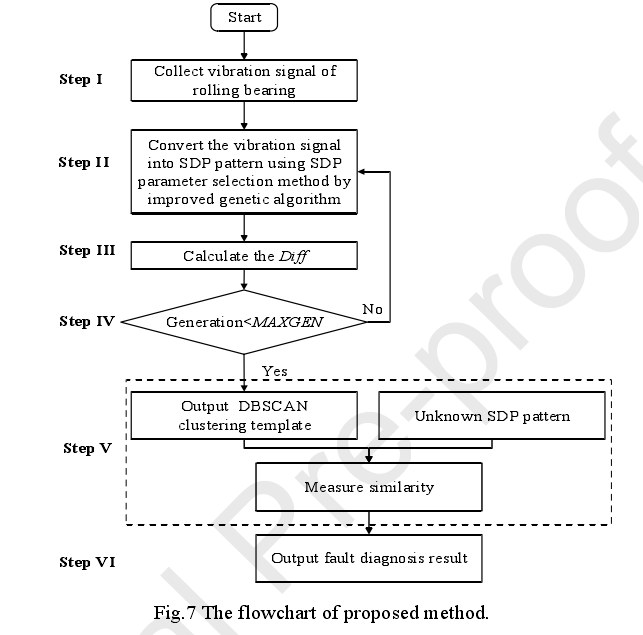SDP:

First give the formula of the drawing: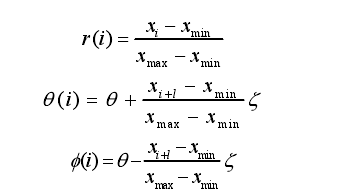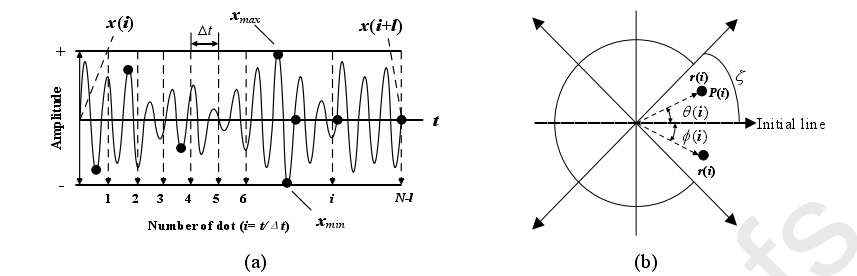In the time domain waveform, the amplitude of the first discrete sample point XI and the amplitude of the i + L sampling point x (i + L) are entered into the SDP formula. Where R (i) is the radius of the pole coordinate, θ (i) is an angle of counterclockwise rotation in a polar coordinate, and φ (i) is an angle that rotates clockwise in a pole coordinate.

L is the maximum and minimum value of the amplitude in the interval of [I, I + L] in the interval parameters, XMAX, respectively. It is assumed that the N such symmetric graphics in a polar coordinate diagram, then θ = 360 m / n (m = 1, 2 … n) θ is a symmetric plane rotation angle, ζ is an amplification factor.

Differences between frequencies are different in polar coordinates and the point distribution position. Three parameters θ,, θ,, θ,, rational selection parameters can increase the resolution of the generated graphics, and it is not easy to overlap.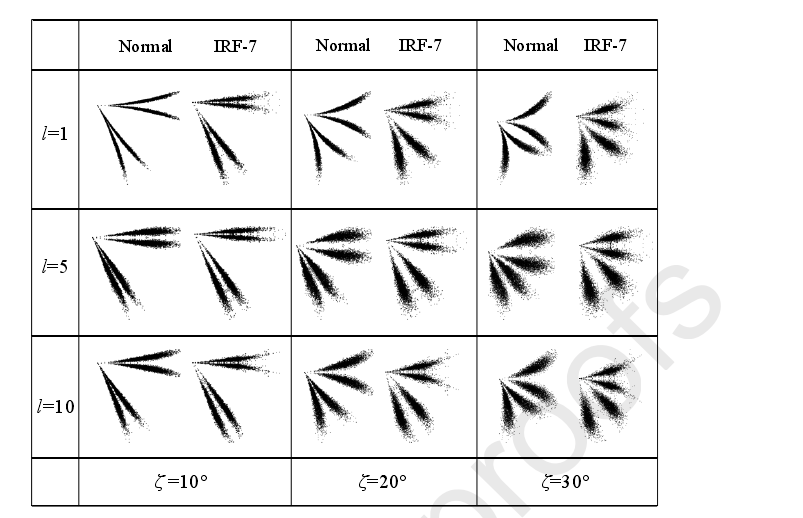As shown in the figure above, when the parameter L = 5 zeta = 10, the normal state and the RF-7 state are very similar, which is not conducive to the machine to identify him. Therefore, this paper proposes a genetic algorithm based on a HILL function from an adaptation to generate parameter values.

First,The digital image processing method converts the SDP mode into a digital matrix.The digital matrix G of the SDP mode can be expressed as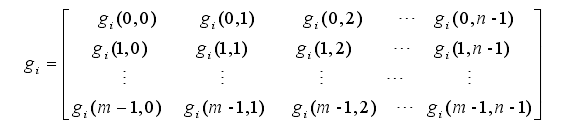Where GI represents a certain working condition (for example, Normal, IRF), each number in the matrix represents the corresponding gradation value on the coordinate axis.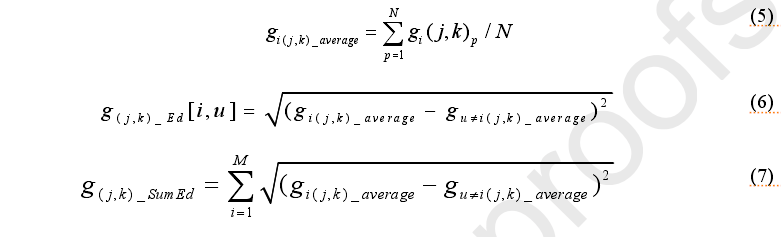For one operational status of the same location, it contains N SDP mode,Equation 5 definesThe average gradation value at (J, K) is GI (J, K) _averageEquation 6 defines the distance between the coordinate positions (J, K) at different working conditions I and U. Equation 7 defines the sum of all operating distances on positions (J, K). It can be concluded by the formula. If the value of the formula 7 is, the larger difference between the SDP image mode, the higher the resolution, the more easily identify classification, extraction characteristics, and the like. His calculation process is as follows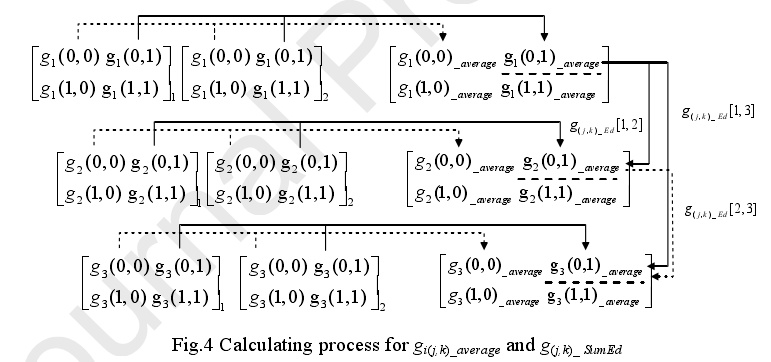Based on the above analysis, finalization of the difference between SDP mode is as follows: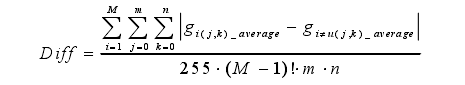In order to obtain the maximum difference, the most suitable L and ζ must be obtained through a search in global solution space.Hill function has a non-negative and monotonicity  (specific document is S. Ko, S. Gunasekaran, Analysis of Cheese Melt Profile Using Inverse-Hill Function, J. Food Eng. 87 (2008) 266-273) Features, for each specific and saturated binding is a good approximation (SATURABLE BINDING translation weird, do not understand what it means).Released by the Hill function, a new probability function P (K) is proposed in EQ. (9) to adjust the scope of the solution, which is beneficial to avoid premature converging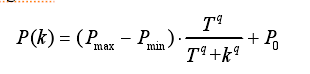​​Among them, the maximum probability PMAX, the minimum probability PMIN, the initial probability P0 is between 0 and 1.The threshold coefficient T and Hill coefficient q are constants throughout the process.K is equal to the current algex, smaller than the maximum algebra MAXGEN.

This can redefine a definition of a genetic algorithm to define cross probability PC (K) and variation probability PM (K)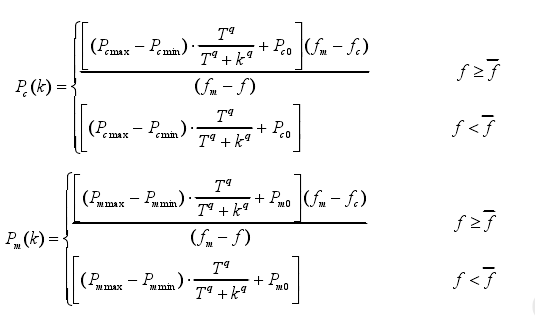The fm is the maximum value of the fit.FC represents a larger adaptivity value when two individuals crossover.f is an individual value of the individual.Pcmax and PMMAX are the maximum value of cross probability and variation probability, respectively.Pcmin and PMMIN are the minimum values ​​of cross probability and variation probability.PM0 and PC0 are the initial values ​​of mutation probability and cross probability, respectively.

DBScan This cluster introduction a few concepts:

Core Object, within a given radius R, the object is more than the object of the Minnum called the core object.

Directly Density-Reachable, there are 2 core objects, one of which is within another range (radius r), is the direct density can be reached

Density-reachable, there is a length of N object sequence, in which X (i + 1) is up to XI, and X1 to XN is Density. .

Density-Connected, 2 objects XI, XJ, there is another object xk, where xk and xi are density, XK and XJ are density, then Xi is connected to XJ, it can be understood Delivered to density.

Clustering Center, in a cluster, the shortest distance from all other core objects.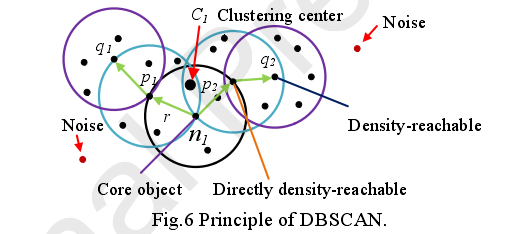,Parameters R and Minnum determine the results of clustering.However, it is difficult to select them reasonably.The density threshold MinNum determines the core object during the cluster, and R determines the search space of the core object.Especially when R is too small, DBSCAN may define useful data as noise.Instead, DBSCAN divides the noise data into a cluster, which may produce a very bad clustering result.For these issues,Improvements proposed in this article DBSCAN: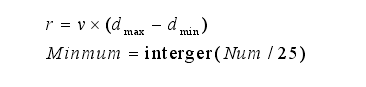V is about the appreciation, the value range is 0 ~ 1.Dmax, Dmin represents the smallest and maximum distance between any two samples, respectively.NUM is the total number of samples.

The method of generating a DBSCan cluster template is as follows (his role is: (That is, test samples) performs differential analysis, if the difference is less than a threshold, match)

Cluster of clustersCluster Centerdbscan clusterTemplateCorresponding coordinate pointThe threshold of the pixel grayscale. andCluster object is a gradation value of the same pixel point under a working condition(Number of grayscale values ​​of the same pixel point = number of SDP images under this working condition. In the later experiment, there are 600 samples under each working condition, and there is a failure of 600 SDP image, then clustering this 600 points to get oneAverageThe grayscale value is excluded from noise), for an operational state I, the digital matrix of the cluster template CI can be represented as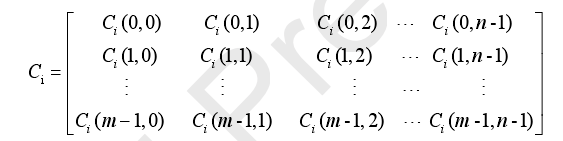(This coordinates on this CI (i, j) template are justGray thresholdThe template should be composed of a point greater than the threshold.

Where CI represents the template matrix of the i-th possible, CI (0,0) represents the cluster center under (0, 0) coordinates, the clustering object comprises Gi (0, 0) 1, GI (0, 0) 2 … GI (0, 0) n, n is the total number of SDP images.

Relevance CC (Correlation Coefficient Definitions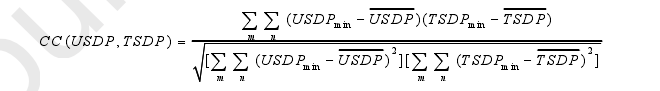The larger the CC value, the more test samples and fault templates.

On the experiment setting,Data acquisition (written by LabVIEW software) The vibration signal is collected on the induction motor and the housing by two PCB 608A11 piezoelectric ICP accelerometers, as shown in FIG.The sampling frequency is set to 8200 Hz, the length of the vibration signal of 0.5S period is 4,100 points.In addition, the experiment of each error will last for 10 minutes.In this study, each measuring point (a accelerometer) contains 4,100 test points, so there are 600 samples per fault.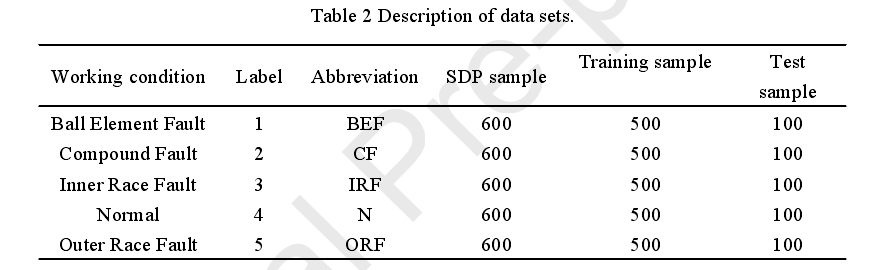The optimal parameter L and are obtained from the genetic algorithm based on the HILL function.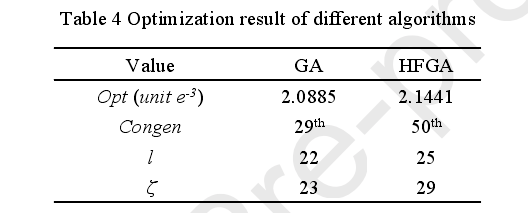Then get the image clustering template of SDP under each working condition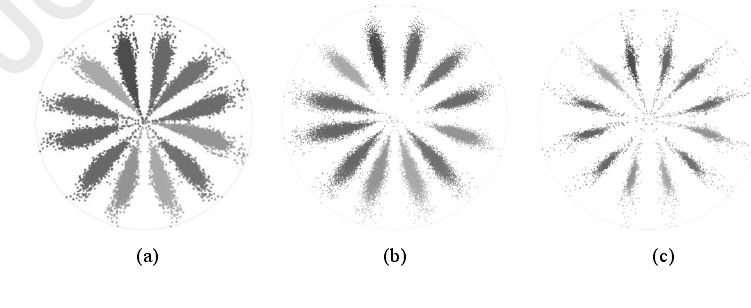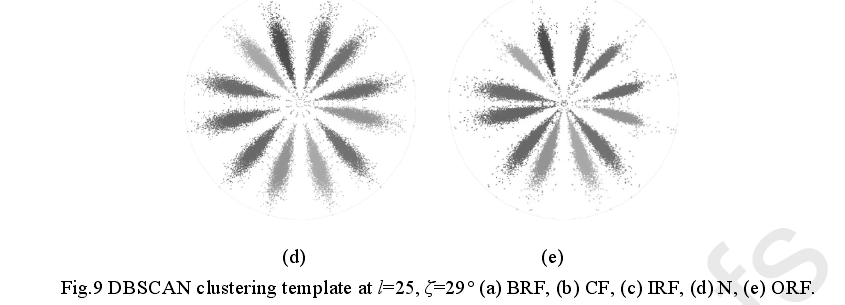After obtaining the DBSCAN cluster template, calculate the correlation coefficient of the unknown SDP image and the cluster template for classification.In order to further demonstrate the advantages of the proposed method (ASDP-DBSCAN), we conducted other four diagnostic methods, including the rut to DBSCAN (Time Domain Extract) , Single Template SDP (Single-SDP) , multi-template SDP (MULTI-SDP) , K-Means cluster SDP (KM-SDP) .Table 5 shows the classification accuracy of different methods.The method is higher than other methods.The results of theASDP-DBSCAN (94.6%) and KURTOSIS-DBSCAN (83.9%) have proved the effectiveness of the SDP-based approach in the field of feature extraction.This may be due to the vibration signal of two different working conditions, and the time domain peak can be the same, thereby generating misuse.Instead, these vibration signals are converted into SDP mode, which may be completely different in the SDP mode.Therefore, the SDP-based method is more correct for the classification results of the same vibration signal.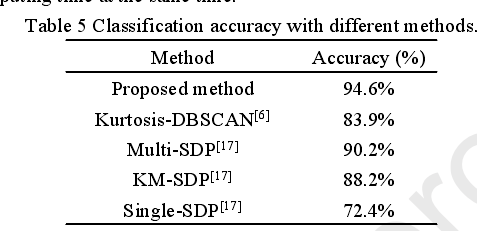Finally, in the case of different noise and different training samples, it also compared, the correct rate of the proposed method is higher than other methods. This is not one here.

Summary:This paper proposes a rolling bearing fault diagnosis method based on adaptive symmetric dot matrix mode analysis and density based clustering method (ASDP-DBSCAN).First, the vibration signal of the rolling bearing operation is analyzed by SDP technology.By analyzing the symmetry mode mode of the vibration signal, the vibration signal can be mapped into an accessible visual representation, and the foundation is laid the foundation for the fault diagnosis.Then, an adaptive SDP parameter selection method is proposed.Combine the Hill function, adaptive update cross probability and variation probability.On this basis, the optimal parameters of the SDP mode are obtained, which is of great significance for reducing the dependence of artificial experience and expertise.Further, an improved DBSCAN is used to generate a fault clustering template to reduce the impact of noise on diagnostic accuracy.Finally, the effectiveness of this method is verified by experiments.Optimization results show that the HFGA algorithm is better than genetic algorithms in search results and search efficiency.In addition, the superiority and effectiveness of the ASDP-DBSCAN method is proved by multiple comparative experiments with other fault diagnosis methods.The experimental results also show that this method has better robustness and effectiveness than Kurtosis-DBSCAN, Multi-SDP, which proves that the method can achieve better performance.

Discussions and Thinking:

1. “Adaptive SDP Parameter Selection Method], in fact, in a given sample, the optimal parameters obtained by training. It feels a bit like static adaptive, because given samples and training, this optimal parameter will not change. If the parameter is changed, only a batch of training status can be used to get a new optimal solution through the genetic algorithm. . It feels not dynamic adaptation. A bit weird

2.Although we use this method for troubleshooting, the generation of cluster templates is a time consuming.For future work, developing a method of reducing calculation time and designing more feature extraction and diagnostic methods is very meaningful.

3.In a latest shed (Mountain big), he cited this article and evaluated “But this type of method is through randomization clustering center, and then the result of continuous iteration”, this I will Not very understanding, the cluster center is not given clearly defined, it feels not random. Random should be the choice or i other parameters of the initial parameters of the adaptive method? Do you want to see a wave again

Words:

Palanetary planetary; Empirical empirical; intrinsic, intrinsic, intrinsic; ensemble, abbreviation abbreviation;

• Top